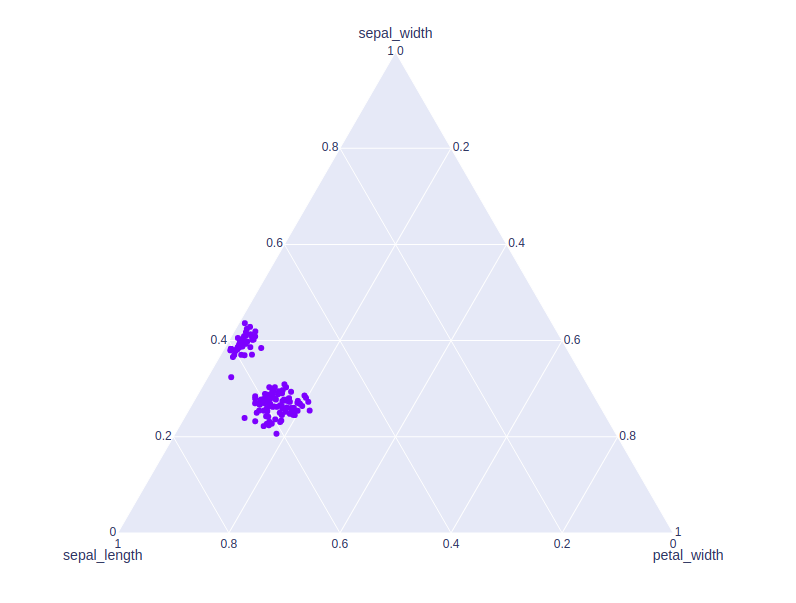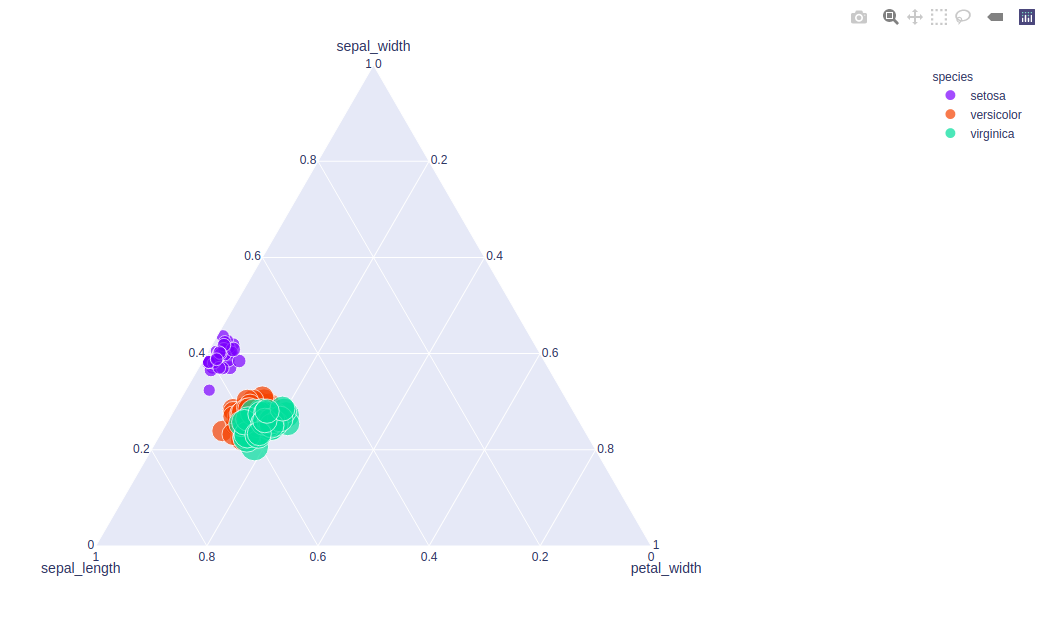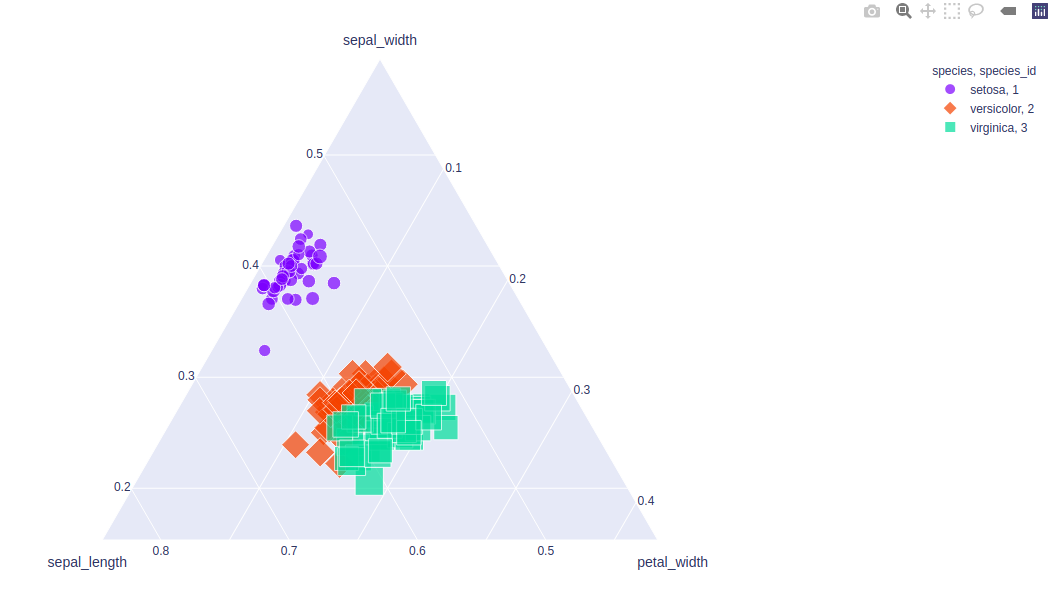Open In App

# plotly.express.scatter_ternary() function in Python

Plotly library of Python can be very useful for data visualization and understanding the data simply and easily. Plotly graph objects are a high-level interface to plotly which are easy to use.

## plotly.express.scatter_ternary() function

This method is used to create a ternary plot. A ternary plot is used to depicts the ratio of three variables on an equilateral triangle.

Syntax: plotly.express.scatter_ternary(data_frame=None, a=None, b=None, c=None, color=None, symbol=None, size=None, text=None, hover_name=None, hover_data=None, size_max=None, title=None, template=None, width=None, height=None)

Parameters:

data_frame: DataFrame or array-like or dict needs to be passed for column names.

a, b, c:  These parameters are either a name of a column in data_frame, or a pandas Series or array_like object. These are used to position marks along the a, b, and c axis in ternary coordinates respectively.

color: This parameters assign color to marks.

symbol: This parameter is used to assign symbols to marks. It is either a name of a column in data_frame, or a pandas Series or array_like object.

size: This parameter is used to assign mark sizes. It is either a name of a column in data_frame, or a pandas Series or array_like object.

hover_name:  Values from this column or array_like appear in bold in the hover tooltip.

hover_data: This parameter is used to appear in the hover tooltip or tuples with a bool or formatting string as first element, and list-like data to appear in hover as second element Values from these columns appear as extra data in the hover tooltip.

title: The title of the figure.

width: Set the width of the figure.

height: Set the height of the figure.

Example 1:

## Python3

 `import` `plotly.express as px` `df ``=` `px.data.iris()` `plot ``=` `px.scatter_ternary(df, a ``=` `'sepal_width'``,``                          ``b ``=` `'sepal_length'``,``                          ``c ``=` `'petal_width'``)``plot.show()`

Output:Example 2: Using the size and color arguments

## Python3

 `import` `plotly.express as px` `df ``=` `px.data.iris()` `plot ``=` `px.scatter_ternary(df, a ``=` `'sepal_width'``,``                          ``b ``=` `'sepal_length'``,``                          ``c``=``'petal_width'``,``                          ``color ``=` `'species'``,``                          ``size ``=` `'petal_length'``)``plot.show()`

Output:Example 3: Using the symbol parameter

## Python3

 `import` `plotly.express as px` `df ``=` `px.data.iris()` `plot ``=` `px.scatter_ternary(df, a ``=` `'sepal_width'``,``                          ``b ``=` `'sepal_length'``,``                          ``c ``=` `'petal_width'``,``                          ``color ``=` `'species'``,``                          ``size ``=` `'petal_length'``,``                          ``symbol ``=` `'species_id'``)``plot.show()`

Output: